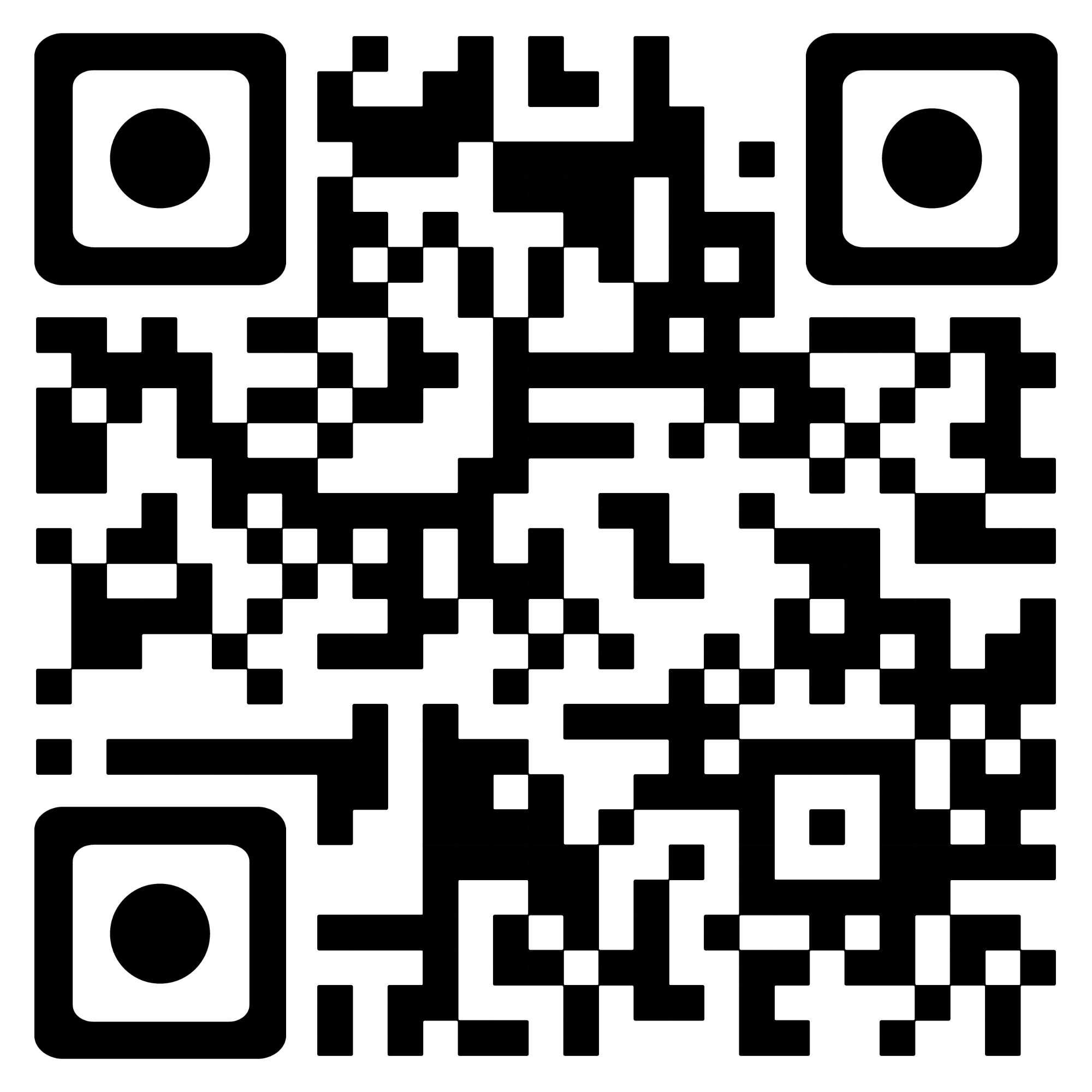Coin Change | Dynamic Programming

You are given an integer array coins representing coins of different denominations and an integer amount representing a total amount of money.

Return the fewest number of coins that you need to make up that amount. If that amount of money cannot be made up by any combination of the coins, return -1.

You may assume that you have an infinite number of each kind of coin.

# Solution

Dynamic Programming

• Still hard for me to understand it ! just fuck it !

# Code

• submit code
``````class Solution:
def coinChange(self, coins: List[int], amount: int) -> int:
self.mem = [-666 for x in range(amount + 1)]
return self.dp(coins,amount)

def dp(self,coins,amount):
if amount == 0:
return 0

if amount < 0:
return  -1

if self.mem[amount] != -666:
return self.mem[amount]

res = 10001
for coin in coins:
subSproblem = self.dp(coins,amount - coin)
if subSproblem == -1:
continue
res = min(res,subSproblem + 1)

self.mem[amount] = -1 if res == 10001 else res
return self.mem[amount]
``````
• full code
``````class SolutionM:
def coinChange(self, coins, amount):
self.arry = [-3 for m in range(amount + 1)]
return self.dp(coins,amount)

def dp(self,coins,amount):
if amount == 0:
return 0

if amount < 0:
return  -1

# create a bak notebook
if self.arry[amount] != -3:
return self.arry[amount]

res = 10001
for coin in coins:
print(coin)
subSproblem = self.dp(coins,amount - coin)
if subSproblem == -1:
continue
res = min(res,subSproblem + 1)

self.arry[amount] = -1 if res == 10001 else res

return self.arry[amount]

S = SolutionM()
coins = [1,2,5]
amount = 1
ret = S.coinChange(coins,amount)

print('-----------------------')
print(ret)
``````

# 👇 Share | 分享 👇

### 要不赞赏一下?微信支付宝PayPalBitcoin

``https://www.emperinter.info/2022/03/16/coin-change/``

## My Github Contributions